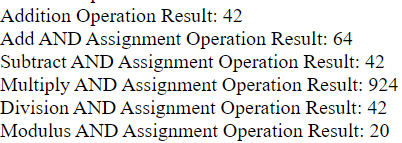# Assignment Operators

## Assignment Operators

PHP uses the (=) to represent the assignment operator. The following shows the syntax of the assignment operator:

\$variable_name = value;

On the left side of the assignment operator (=) is a variable to which you want to assign a value. And on the right side of the assignment operator (=) is a value.

Assignment by reference is also supported, using the "\$var = &\$othervar;" syntax. Assignment by reference means that both variables end up pointing at the same data, and nothing is copied anywhere.

\$var = 5;

\$var1 = &\$var; // \$var1 is a reference to \$var

### Arithmetic Assignment Operators

 Example Equivalent Operation \$a += \$b \$a = \$a + \$b Addition \$a -= \$b \$a = \$a - \$b Subtraction \$a *= \$b \$a = \$a * \$b Multiplication \$a /= \$b \$a = \$a / \$b Division \$a %= \$b \$a = \$a % \$b Modulus \$a **= \$b \$a = \$a ** \$b Exponentiation

Example

<?PHP

\$a = 22; //Assign Value to a.

\$b = 20; //Assign Value to b.

\$Sum = \$a + \$b;

echo "Addition Operation Result: \$Sum <br/>";

\$Sum += \$a;

echo "Add AND Assignment Operation Result: \$Sum <br/>";

\$Sum -= \$a;

echo "Subtract AND Assignment Operation Result: \$Sum <br/>";

\$Sum *= \$a;

echo "Multiply AND Assignment Operation Result: \$Sum <br/>";

\$Sum /= \$a;

echo "Division AND Assignment Operation Result: \$Sum <br/>";

\$Sum %= \$a;

echo "Modulus AND Assignment Operation Result: \$Sum <br/>";

?>

The result of the above code is:### Bitwise Assignment Operators

 Operation Example Equivalent And Assignment (&=) \$a &= \$b \$a = \$a & \$b Or Assignment (|=) \$a |= \$b \$a = \$a | \$b Xor Assignment (^=) \$a ^= \$b \$a = \$a ^ \$b Left Shift Assignment (<<=) \$a <<= \$b \$a = \$a << \$b Right Shift Assignment (>>=) \$a >>= \$b \$a = \$a >> \$b

Example:

<?php
\$x = 19;
\$x &= 7;
\$result= \$x;
echo "And Assignment Result: \$result <br/>";
\$y = 19;
\$y |= 7;
\$result= \$y;
echo "Or Assignment Result: \$result <br/>";
\$z = 19;
\$z ^= 7;
\$result= \$z;
echo "Xor Assignment Result: \$result <br/>";
\$f = 7;
\$f <<= 2;
\$result= \$f;
echo "Right Shift Assignment Result: \$result <br/>";
\$g = 19;
\$g >>= 2;
\$result= \$g;
echo "Right Shift Assignment Result: \$result <br/>";

?>